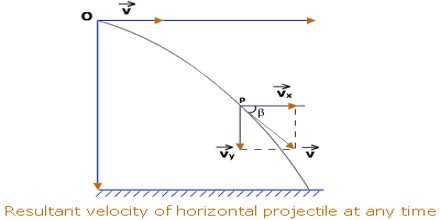Physics

# Equation of Motion of a Horizontal ProjectileEquation of motion of a horizontal projectile

Let an object be thrown from a point O with velocity v0 along the horizontal direction [Figure]. If we ignore air resistance and consider g constant, then along the path of motion of the thrown object v0 will remain constant. Let after t sec the object reach at point P travelling distances x and y, respectively along horizontal and vertical directions. Now let the velocity at P be v. The horizontal and vertical components of v are respectively vx and vy.So, vx = v0 = v cos θ

and vy = v0 + gt = 0 + gt = v + sin θ

[as we know, v0 = 0 along vertical direction]

so, v = √(vx2 + vy2)

here angle θ in between v and horizontal direction, i.e., along X-asis,

and, tan θ = vy/vx

Again, x = v0 + t … … … (1)

[ax = 0 along horizontal direction]

so, t = x/v0

and, y = ½ gt2 …. …. …. (2)

[v0 = 0 along vertical direction]

Now, inserting the value of t from equation (1) in equation (2) we get,

y = ½ g(x/v0)2

so, x2 = (2v02/g).y … … … (3)

Since, v0 and g are constant, if we put 2v02/g = 4A, we get,

x2 = 4A.y

This is an equation of parabola. So, trajectory of a body or a projectile ejected horizontally in resistant free-path forms a parabola.